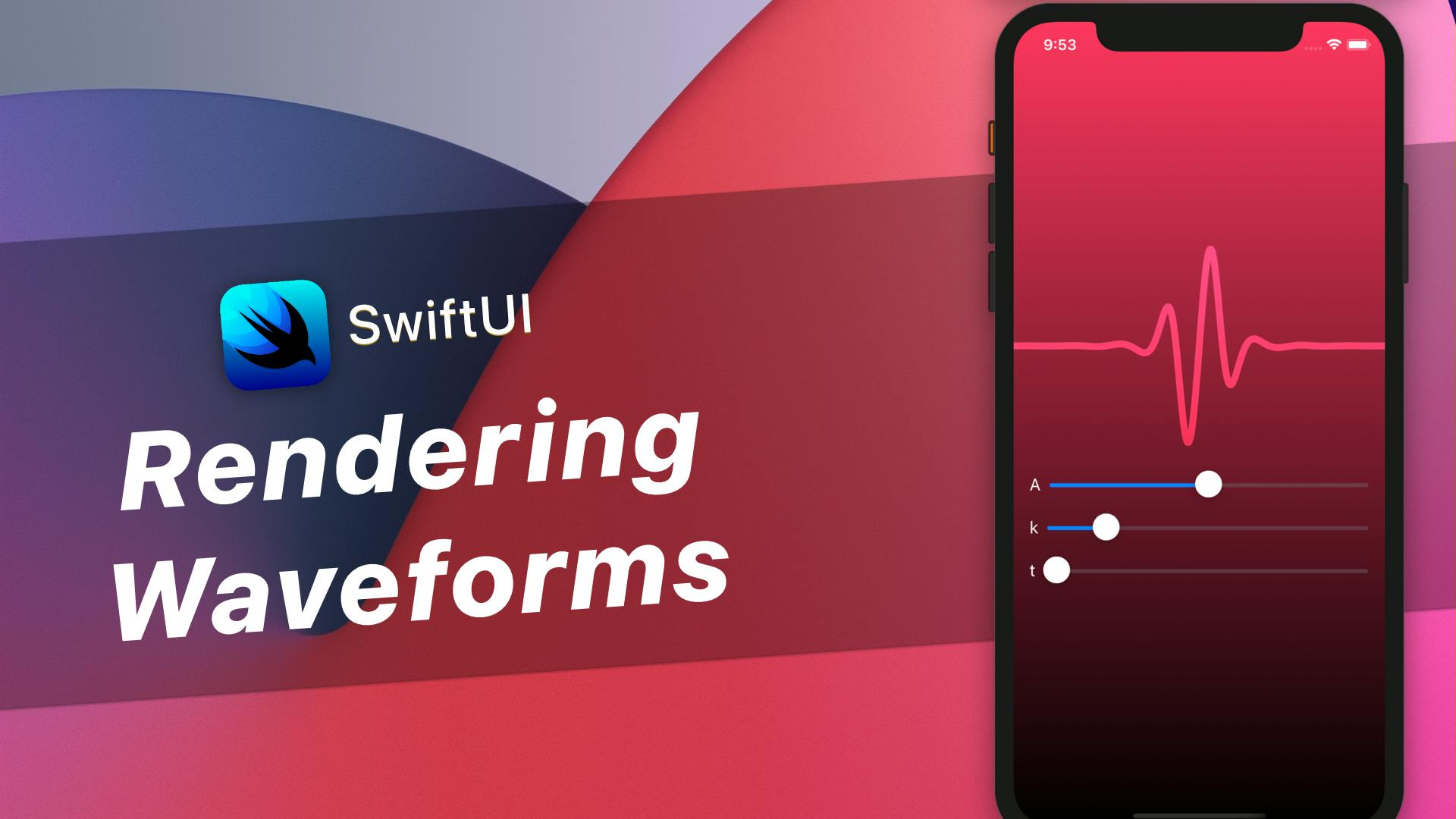Episode #447

# Rendering Waveforms in SwiftUI

28 minutes
Published on July 9, 2020## This video is only available to subscribers.Get access to this video and 507 others.

I've been working on rendering waveforms using mathematical functions and have found the experience to be both fun and enlightening. In this episode we will develop a method to render arbitrary functions using a Shape, then explore some mathematical concepts that can help us render a nice looking waveform that could be use to indicate activity in sound, speech, or other effects.

How I built the SiriWaveJS library - The math included in this episode was inspired by this blog post, where Flavio De Stafano renders the original Siri waveform using JavaScript. I found this post to be incredibly helpful in understanding the concepts.

## Defining the WaveForm Shape

First we define a shape we can use which takes a function that will define the line we'll plot.

``````struct WaveFormShape: Shape {

let fn: (Double) -> Double = { _ in 0 }
let range: ClosedRange<Double> = -1...1
let steps: Int = 3

func path(in rect: CGRect) -> Path {
...
}
}
``````

Next we define a computed property to render each point:

``````    var points: [CGPoint] {
var points = [CGPoint]()
let xStride = (range.upperBound-range.lowerBound) / Double(steps-1)
for x in stride(from: range.lowerBound, through: range.upperBound, by: xStride) {
let y = fn(x)
let p = CGPoint(x: x, y: y)
points.append(p)
}

return points
}
``````

This gives us an array of graph points. Next we need to apply this to our view space, and for that we'll need the rect to transform these points into:

``````    private func normalizedPoints(in rect: CGRect) -> [CGPoint] {
let points = self.points
return points.enumerated().map { (offset, p) in
let screenX = CGFloat(offset) * rect.width/CGFloat(points.count - 1)
let screenY = rect.midY - (p.y * rect.height/2)
return CGPoint(x: screenX, y: screenY)
}
}
``````

Here we define the `y` range with 0 being in the middle of the graph, 1 at the top, and -1 at the bottom.

Finally we can implement our path function:

``````    func path(in rect: CGRect) -> Path {
Path { p in
let points = normalizedPoints(in: rect)
}
}
``````

## Graphing a Tapered Sine Curve

``````struct GraphView: View {

var amplitude: Double = 2
var frequency: Double = 4
var phase: Double = 0

var body: some View {
...
}
}
``````

Then we define the function that we want to use. For this I'll define a configurable sine function:

``````    private func sineFunc(_ x: Double) -> Double {
amplitude * sin(frequency * x - phase)
}
``````

As well as a windowing or "tapering" function we can use to filter out values on the edges:

``````    private func taperFunc(_ x: Double) -> Double {
let K: Double = 1
return pow(K/(K + pow(x, 4)), K)
}
``````

Now we can define the body of our view, rendering the waveform shape with the above functions:

``````        WaveForm(
fn: { sineFunc(\$0) * taperFunc(\$0) },
steps: 300,
range: (-2 * .pi)...(2 * .pi)
)
.stroke(Color.white, style:
StrokeStyle(lineWidth: 7, lineCap: .round, lineJoin: .round, miterLimit: 10, dash: [], dashPhase: 0)
)
``````

## Displaying a tweakable interface

To show this graph with some configurable sliders, we'll create some bindings we can pass in and control with sliders.

``````struct ContentView: View {
@State var amplitude: Double = 1.0
@State var frequency: Double = 4.0
@State var phase: Double = 0

var body: some View {
ZStack {
VStack {

GraphView(amplitude: amplitude, frequency: frequency, phase: phase)
.frame(height: 200)
.blendMode(.overlay)

VStack {
ParamSlider(label: "A", value: \$amplitude, range: 0...2.0)
ParamSlider(label: "k", value: \$frequency, range: 1...20)
ParamSlider(label: "t", value: \$phase, range: 0...(.pi * 40))
}
}.edgesIgnoringSafeArea(.all)
}
}
``````

Here we use a simple extension on `LinearGradient` to give it a nice background:

``````extension LinearGradient {
}
``````

And we also use a simple `ParamSlider` component to simplify the label, slider, and range for each of our parameters:

``````struct ParamSlider: View {
var label: String
var value: Binding<Double>
var range: ClosedRange<Double>

var body: some View {
HStack {
Text(label)
Slider(value: value, in: range)
}
}
}
``````

This episode uses Swift 5.3, Ios 14.0-beta2, Xcode 12-beta2.